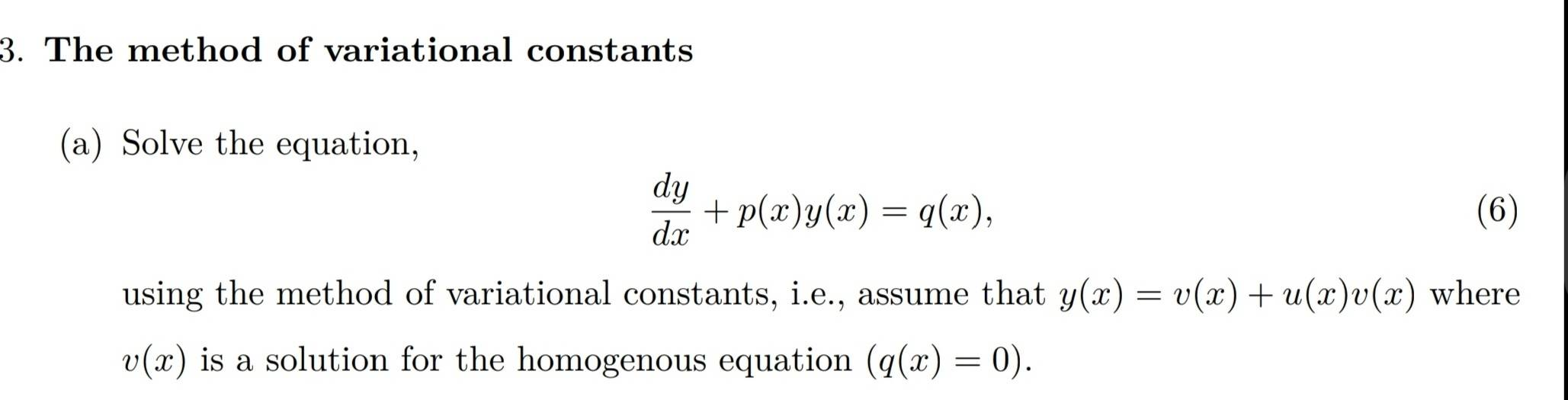# First order differential equations

• MHB
• Bat1
In summary, the given equation is separable and has a general solution of y(x)= Ce^{-\int p(x)dx}. To find a solution to the entire equation, we use the method of variation of parameters, looking for a solution of the form y(x)= u(x)e^{-\int p(x)dx}. This leads to the equation u'= \frac{q(x)}{v(x)}= \frac{q(x)}{e^{-\int p(t)dt}}, which can be solved for u(x) by integration by parts. The final solution is y(x)= u(x)e^{-\int p(x)dx}.

#### Bat1

Hi,
y(x) _homogenous =v(x)
y(x) _private =u(x)v(x)
?
Or they refer to something else?
I don't know how to approach to itThe given equation is $$\displaystyle \frac{dy}{dx}+ p(x)y(x)= q(x)$$. The "associated homogeneous equation" is $$\displaystyle \frac{dy}{dx}+ p(x)y(x)= 0$$. (As they say, "q(x)= 0").

That equation is "separable". $$\displaystyle \frac{dy}{dx}= -p(x)y(x)$$ and then $$\displaystyle \frac{dy}{y}= -p(x)dx$$.

Integrating both sides the general solution to the associated homogeneous equation is $$\displaystyle ln(y(x))= -\int p(x)dx+ c$$.
Take the exponential of both sides- $$\displaystyle y(x)= e^{-\int p(x)dx+ c}= Ce^{-\int p(x)dx}$$ where $$\displaystyle C= e^c$$.

Now, they are saying that you should look for a solution of the form "y(x)= v(x)+ u(x)v(x) where v(x) is a solution of the homogenous equation" (I would call this method "variation of parameters" rather than "variable parameters" also, since v(x) satisfies the homogenous equation, putting it alone into the equation will give 0 so I would use just y(x)= u(x)v(x). ) so $$\displaystyle y(x)= u(x)e^{-\int p(t)dt}$$).

Then $$\displaystyle y'= u'e^{-\int p(x)dx}- u(x)\left(p(x)e^{-\int p(x)dx}\right)= u'v- p(x)u(x)v$$ and $$\displaystyle y'+ p(x)y= u'v- p(x)u(x)v+ p(x)u(x)v= u'v= q(x)$$. So $$\displaystyle u'= \frac{du}{dx}= \frac{q(x)}{v(x)}=$$$$\displaystyle \frac{q(x)}{e^{-\int p(t)dt}}$$ and $$\displaystyle u(x)= \int \frac{q(x)}{e^{-\int p(t)dt}}dx$$.

For a very simple example, consider $$\displaystyle y'- 2y= x^2$$. p(x) is the constant -2 and q(x) is $$\displaystyle x^2$$.

The associated homogeneous equation is $$\displaystyle y'- 2y= 0$$ or $$\displaystyle y'= \frac{dy}{dx}= 2y$$ which can be separated as $$\displaystyle \frac{dy}{y}= 2dx$$. Integrating $$\displaystyle ln(y)= 2x+ c$$ and, solving for y, $$\displaystyle y(x)= Ce^{2x}$$.

Now, using "variational constants" we look for a solution to the entire equation of the form $$\displaystyle y(x)= u(x)e^{2x}$$. Then $$\displaystyle y'= u'e^{2x}+ 2ue^{2x}$$ so the equation becomes $$\displaystyle y'- 2y= u'e^{2x}+ 2ue^{2x}- 2ue^{2x}= u'e^{2x}= x^2[/tex$$.

$$\displaystyle u'= \frac{du}{dx}= x^2e^{-2x}$$ so $$\displaystyle u= \int x^2 e^{-2x}dx$$.

To integrate that, use "integration by parts, taking $$\displaystyle u= x^2$$, so that $$\displaystyle du= 2xdx$$, and $$\displaystyle dv= e^{-2x}dx$$ so that $$\displaystyle v= -\frac{1}{2}e^{-2x}$$.

$$\displaystyle \int x^2e^{-2x}dx= -\frac{1}{2}x^2e^{-2x}+ \int xe^{-2x}dx$$.

To integrate $$\displaystyle \int xe^{-2x}dx$$ use "integration by parts" again this time with $$\displaystyle u= x$$ so that $$\displaystyle du= dx$$and $$\displaystyle dv= e^{-2x}dx$$ so that $$\displaystyle v= -\frac{1}{2}e^{-2x}$$.

$$\displaystyle \int xe^{-2x}dx= -\frac{1}{2}xe^{-2x}+ \frac{1}{2}\int e^{-2x}dx= -\frac{1}{2}xe^{-2x}- \frac{1}{4}e^{-2x}+ C$$.